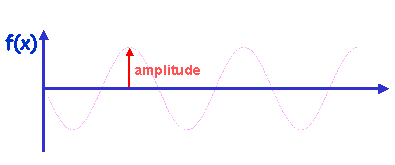## Amplitude

• The amplitude of a wave is the peak value (i.e. the height) of the wave in the positive or negative direction.For example, for a general sine wave:

f(t) = A sin(Bt), where A = amplitude of the wave
T = 2π/B = period, and
f = 1/T = B/2π = frequency